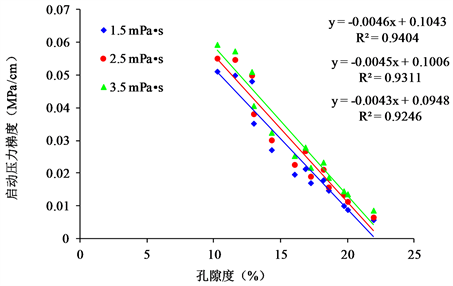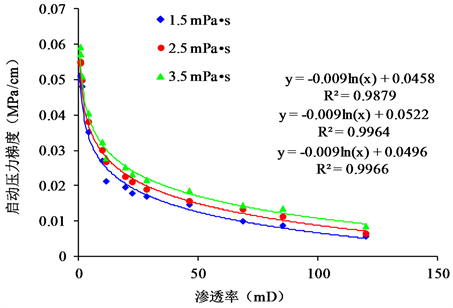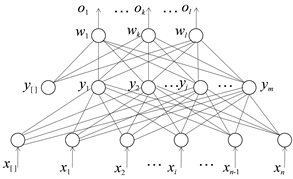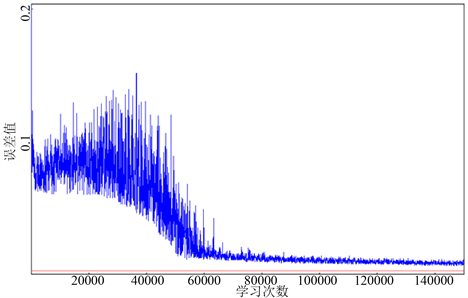﻿ 东濮老油田注水井分层启动压力预测技术研究

# 东濮老油田注水井分层启动压力预测技术研究Study on Stratified Starting Pressure Prediction Technology for Injection Wells in Dongpu Mature Oilfield

Abstract: The stratified starting pressure is the main basis to determine the stratified water injection program. Based on the reservoir characteristics of Dongpu mature area of Zhongyuan oilfield, this paper studies the variation rule of starting pressure gradient with reservoir physical property. A small layer startup pressure prediction model based on BP neural network is established. A set of starting pressure calculation method suitable for reservoir characteristics is formed. The method is of great significance for guiding fine water injection and improving the effect of water injection development.

1. 前言

2. 启动压力梯度实验研究Figure 1. Chart of relationship between starting pressure gradient and porosityFigure 2. Chart of relationship between starting pressure gradient and permeability

3. 注水小层启动压力影响因素研究

4. 注水小层启动压力预测方法研究

4.1. BP神经网络

BP神经网络是一种按误差反向传播(误差反传)训练的多层前馈网络  (见图3)。BP算法包括信号的正向传播和误差的反向传播  两个过程，即计算误差输出时按从输入到输出的方向进行，而调整权值和阈值则从输出到输入的方向进行。输入层各神经元接收来自外界的输入信息，并传递给中间层各神经元，而中间层负责信息转换，误差通过输出层，按误差梯度下降的方式修正各单元权值，向隐层、输入层逐层反传 。经过反复学习训练，神经网络即能对类似样本的输入信息自行处理输出误差最小的经过非线形转换的信息(见图4)。Figure 3. Structure of BP networkFigure 4. Three layer BP network diagram

${o}_{k}=f\left(ne{t}_{k}\right),\text{\hspace{0.17em}}\text{\hspace{0.17em}}k=1,2,\cdots ,l$ (1)

$ne{t}_{k}=\underset{j=0}{\overset{m}{\sum }}{w}_{jk}{y}_{j},\text{\hspace{0.17em}}\text{\hspace{0.17em}}k=1,2,\cdots ,l$ (2)

${y}_{j}=f\left(ne{t}_{j}\right),\text{\hspace{0.17em}}\text{\hspace{0.17em}}j=1,2,\cdots ,m$ (3)

$ne{t}_{j}=\underset{i=0}{\overset{n}{\sum }}{v}_{ij}{x}_{i},\text{\hspace{0.17em}}\text{\hspace{0.17em}}j=1,2,\cdots ,m$ (4)

$f\left(x\right)=\frac{1}{1+{\text{e}}^{-x}}$ (5)

$E=\frac{1}{2}{\left(d-O\right)}^{2}=\frac{1}{2}\underset{k=1}{\overset{l}{\sum }}{\left({d}_{k}-{o}_{k}\right)}^{2}$ (6)

$E=\frac{1}{2}\underset{k=1}{\overset{l}{\sum }}{\left[{d}_{k}-f\left(ne{t}_{k}\right)\right]}^{2}=\frac{1}{2}{\underset{k=1}{\overset{l}{\sum }}\left[{d}_{k}-f\left(\underset{j=0}{\overset{m}{\sum }}{w}_{jk}{y}_{j}\right)\right]}^{2}$ (7)

$E=\frac{1}{2}{\underset{k=1}{\overset{l}{\sum }}\left\{{d}_{k}-f\left[\underset{j=0}{\overset{m}{\sum }}{w}_{jk}f\left(ne{t}_{j}\right)\right]\right\}}^{2}=\frac{1}{2}{\underset{k=1}{\overset{l}{\sum }}\left\{{d}_{k}-f\left[\underset{j=0}{\overset{m}{\sum }}{w}_{jk}f\left(\underset{i=0}{\overset{n}{\sum }}{v}_{ij}{x}_{i}\right)\right]\right\}}^{2}$ (8)

$\Delta {w}_{jk}=-\eta \frac{\partial E}{\partial {w}_{jk}},\text{\hspace{0.17em}}\text{\hspace{0.17em}}j=0,1,2,\cdots ,m;\text{\hspace{0.17em}}\text{\hspace{0.17em}}k=1,2,\cdots ,l$ (9)

$\Delta {v}_{ij}=-\eta \frac{\partial E}{\partial {v}_{ij}},\text{\hspace{0.17em}}\text{\hspace{0.17em}}j=0,1,2,\cdots ,n;\text{\hspace{0.17em}}\text{\hspace{0.17em}}k=1,2,\cdots ,m$ (10)

4.2. 分层启动压力预测Figure 5. Variation of network error with training timesTable 1. Network training parameters and overall relative error

Table 2. Experimental data and prediction results

5. 结论

1) 结果表明：基于BP神经网络，建立综合考虑启动压力梯度、储层特性及注水条件等因素的注水小层启动压力预测技术是可行和可靠的，适用于同类油藏在没有测试资料的条件下确定各小层启动压力，可以为分层治理提供决策依据，有效指导老区精细注采，提高水驱动用程度。

2) 应用区块样本数据的准确性对于启动压力预测数据的精度有着直接的关系，建议结合油藏地质特性建立相关物性参数模型，以保证样本数据的准确性，提高小层启动压力预测的精度。

3) 分层启动压力的影响因素较多，这些因素之间又存在一定的关联，并且不同地区各影响因素之间的关联性不是一成不变的，针对目标油藏的影响因素分析及小层启动压力预测有待进一步研究和完善。

 罗庆, 李晓蕾, 等. 氧活化分层启动压力测试资料在中原油田的应用[J]. 内蒙古石油化工, 2016(10): 40-41.

 荣准, 杨大良, 等. 注水井分层启动压力预测方法研究[J]. 中国石油化工标准与质量, 2012(10): 184-188.

 王亚雄. 分层启动压力测试技术应用[J]. 中国石油石化, 2016(z2): 22-23.

 田巍, 朱维耀, 朱华银, 等. 致密砂岩启动压力梯度数值的影响因素[J]. 断块油气田, 2014, 21(5): 611-614.

 吕成远, 王建, 孙志刚. 低渗透砂岩油藏渗流启动压力梯度实验研究[J]. 石油勘探与开发, 2002, 29(2): 86-89.

 聂玲, 周德胜, 郭向东, 惠建军, 边疆, 高利军. 利用灰色关联法分析低渗气藏压裂影响因素[J]. 断块油气田, 2013, 20(1): 133-136.

 任双双, 杨胜来, 沈飞. BP神经网络预测最小混相压力[J]. 断块油气田, 2010, 17(2): 216-218.

 朱长军, 郝振纯, 等. 启动压力梯度预测的人工神经网络方法[J]. 西安石油大学学报(自然科学版), 2006, 21(5): 65-67.

 马海志. BP神经网络的改进研究及应用[D]: [硕士学位论文]. 哈尔滨: 东北农业大学, 2015.

 周晋冲, 韩晓冰, 等. BP神经网络在启动压力梯度预测中的应用[J]. 中国化工贸易, 2017, 9(1): 115-116.

Top